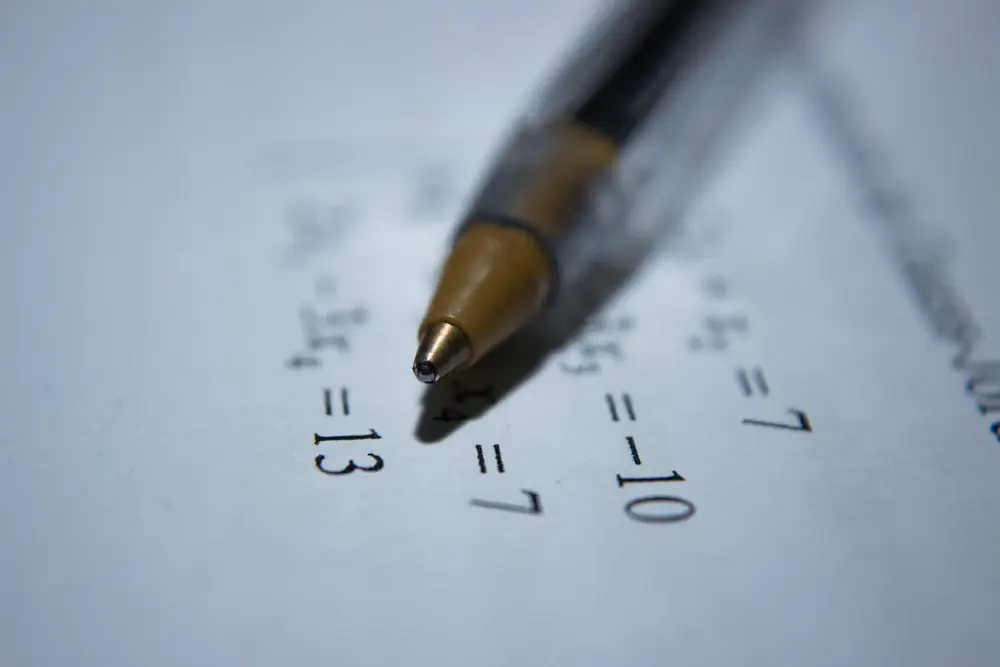# Reduction to linear form

• In order to show that data from a science experiment fits a rule, we have to be able to plot two variables on a graph so that a straight line relationship results (this will be the line of best fit if the data is experimental).
• In questions, you may be given some data and you may be asked to plot y against 1/x . If the data lies in a straight line, you know, from the equation of a straight line (y = mx + c), that y = m/x + c, since 1/x is on the x-axis. You have therefore found the relationship between the data.
• Example:
• V 5 10 15 20 25
• R 149 175 219 280 359
• By drawing a graph, show that these pairs of values may be regarded as approximations to values satisfying a relationship of the form R = a + bV² .
• In this question, you would plot R against V² . If a straight line comes out, then you know that (from y = mx + c) R = mV² + c (which is just the same as R = a + bV², since m and c, a and b are just constants).
• The second part of such a question may ask you to calculate the values of a and b. In this example, b is the gradient of the graph and a is the y-intercept (this can again be established by comparing the equation to y = mx + c).
Algebra

Algebra

Algebra

Algebra

Algebra

Algebra